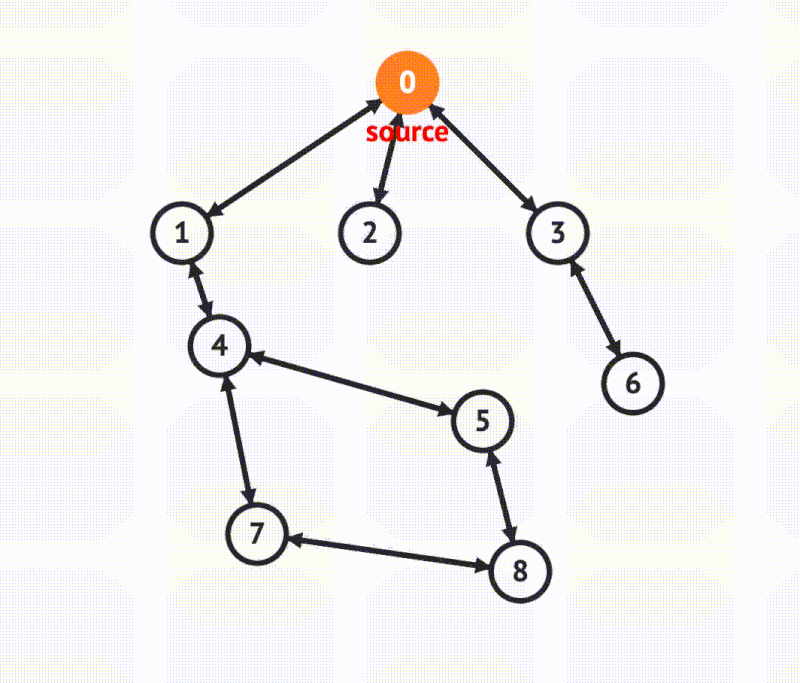Depth-First Search | Skilled.dev
Learn

# Depth-First SearchCredit: VisuAlgo
Pros 🔥
• Less memory used
We often assume paths tend to contain less nodes than the width, so DFS is typically better with memory than BFS. DFS is better if the graph is wider than it is deep.
• Determine if a path exists
It is efficient in finding if a path between a source and target exists.
Cons 🤕
• Deep graphs are slow
DFS can get slow if the graph is deep because it follows paths fully before moving to the next. BFS outperforms DFS when graphs are deeper than they are wide.

Depth-first search (DFS) follows paths to their end before moving on to the next path. It goes deep before it goes wide.

Key concepts:

• Use a stack (recursive call stack or an actual stack data structure in your code)
• Go deep before wide (follow a path to its end)
• Track visited nodes to avoid cycles or multiple visits

I highly recommend watching the video to visualize DFS, understand why we use a stack, and gain a strong intuition with how it works.

To implement DFS, we use a stack data structure. With recursive DFS, our stack is just the function call stack, but if we do it iteratively, we create an actual stack data structure in our code. A stack is used because we continue to add items to it until we reach the end of a path. Then we pop them off until we return to the beginning of the path and perform the operation again on the next path.

DFS is often assumed to require less memory than BFS because the width of a path tends to have more nodes than its height. DFS only stores a path whereas BFS must store all of a node's neighbors. Because of this assumption on less memory, DFS is typically the preferred choice.

To implement DFS, we will use a graph node that stores `data` and its `edges`. The `edges` variable is just an array of pointers to its sibling nodes. We will also assume our graph is undirected.

Nodes will take the following form:

`` ``

Because there can be cycles in graphs or multiple nodes that share an edge, we need to track if we have visited a given item. If we don't track this, we could get stuck in an infinite loop and traverse our graph indefinitely.

You can interact with this code in a REPL, and the iterative DFS solution is given below:

`` ``

In the iterative solution, we maintain our stack and track if we have visited a node. By using a stack, we are always traversing from the most recently added item (LIFO) which keeps us moving towards the end of any given path.

The recursive DFS solution is below. In this case, the stack used to store nodes is actually the call stack.

`` ``
Ready for the next step?
Find a Job